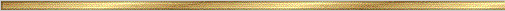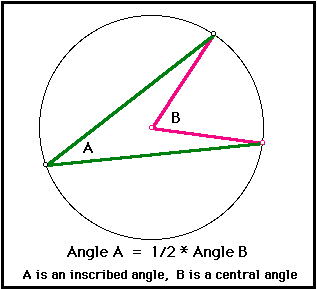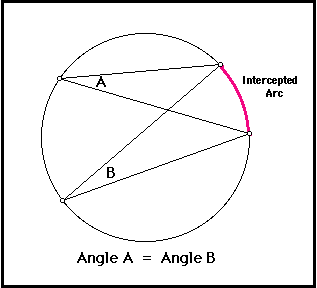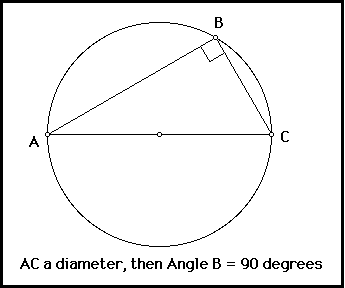# Inscribed Angles Conjectures### Explanation:

An inscribed angle is an angle formed by two chords in a circle which have a common endpoint. This common endpoint forms the vertex of the inscribed angle. The other two endpoints define what we call an intercepted arc on the circle. The intercepted arc might be thought of as the part of the circle which is "inside" the inscribed angle. (See the pink part of the circle in the picture above.)

A central angle is any angle whose vertex is located at the center of a circle. A central angle necessarily passes through two points on the circle, which in turn divide the circle into two arcs: a major arc and a minor arc. The minor arc is the smaller of the two arcs, while the major arc is the bigger. We define the arc angle to be the measure of the central angle which intercepts it.

The Inscribed Angle Conjecture I gives the relationship between the measures of an inscribed angle and the intercepted arc angle. It says that the measure of the intercepted arc is twice that of the inscribed angle.

The precise statements of the conjectures are given below. Each conjecture has a linked Sketch Pad demonstration to illustrate its truth (proof by Geometer's Sketch Pad!). The linked activities sheet also include directions for further "hands on" investigations involving these conjectures, as well as geometric problems which utilize their results.

### The precise statement of the conjectures:

Conjecture (Inscribed Angles Conjecture I ): In a circle, the measure of an inscribed angle is half the measure of the central angle with the same intercepted arc..Corollary (Inscribed Angles Conjecture II ): In a circle, two inscribed angles with the same intercepted arc are congruent.Proof: The measure of each inscribed angle is exactly half the measure of its intercepted arc. Since they have the same intercepted arc, they have the same measure.

Corollary (Inscribed Angles Conjecture III ): Any angle inscribed in a semi-circle is a right angle.Proof: The intercepted arc for an angle inscribed in a semi-circle is 180 degrees. Therefore the measure of the angle must be half of 180, or 90 degrees. In other words, the angle is a right angle.